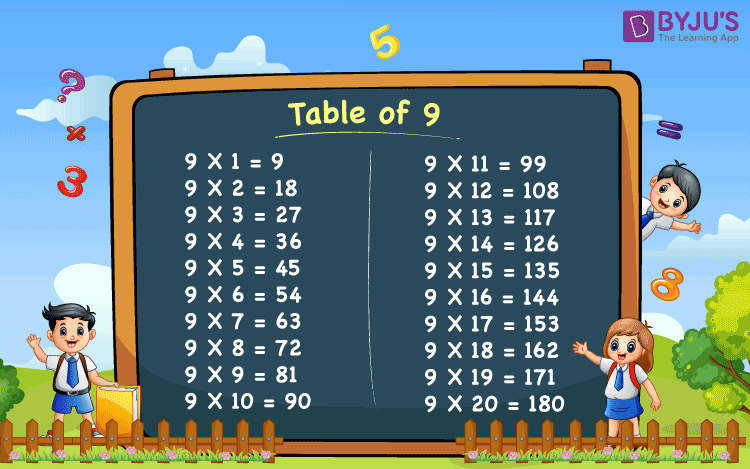# Table of 9

Table of 9 is provided here. Students who are studying in class 5 should try to memorise the table. Memorising the 9 times table will help the students in fast multiplications and time-based examinations where the number of questions is more than the time allotted to the examination. It is recommended to everyone that they should learn the multiplication table given below:

### 9 Times Table Chart### What is 9 times table?

The table of 9 can be described using the repeated addition of 9. For example, a basket has 6 different colours of 9 balls each. In this case, the total number of balls in the basket can be calculated using the multiplication table.

That means each colour contains 9 balls.

Thus, the total number of balls = 9 × 6 = 54

This can also be expressed as, 9 + 9 + 9 + 9 + 9 + 9 = 54

Hence, we can get all the multiples of 9 as explained above.

By adding 9’s we can write the 9 table from 1 to 10 as:

 Nine times table up to 10 9 × 1 = 9 9 9 × 2 = 18 9 + 9 = 18 9 × 3 = 27 9 + 9 + 9 = 27 9 × 4 = 36 9 + 9 + 9 + 9 = 36 9 × 5 = 45 9 + 9 + 9 + 9 + 9 = 45 9 × 6 = 54 9 + 9 + 9 + 9 + 9 + 9 = 54 9 × 7 = 63 9 + 9 + 9 + 9 + 9 + 9 + 9 = 63 9 × 8 = 72 9 + 9 + 9 + 9 + 9 + 9 + 9 + 9 = 72 9 × 9 = 81 9 + 9 + 9 + 9 + 9 + 9 + 9 + 9 + 9 = 81 9 × 10 = 90 9 + 9 + 9 + 9 + 9 + 9 + 9 + 9 + 9 + 9 = 90

The above table (9 table up to 10) shows the first 10 multiples of 9.

## Multiplication Table of 9

The multiplication table of 9 from 1 to 20 is given below:

 9 X 1 = 9 9 X 2 = 18 9 X 3 = 27 9 X 4 = 36 9 X 5 = 45 9 X 6 = 54 9 X 7 = 63 9 X 8 = 72 9 X 9 = 81 9 X 10 = 90 9 X 11 = 99 9 X 12 = 108 9 X 13 = 117 9 X 14 = 126 9 X 15 = 135 9 X 16 = 144 9 X 17 = 153 9 X 18 = 162 9 X 19 = 171 9 X 20 = 180

### How to Read Table of 9?

Read the nine times table from 1 to 10 as provided below.

• One time nine is nine
• Two times nine is eighteen
• Three times nine is twenty-seven
• Four times nine is thirty-six
• Five times nine is forty-five
• Six times nine is fifty-four
• Seven times nine is sixty-three
• Eight times nine is seventy-two
• Nine times nine is eighty-one
• Ten times nine is ninety

### Tips to Memorize the Nine times table

We can easily remember the nine times table from 1 to 10.

Tip 1:

The first 10 multiples of 9 are: 09, 18, 27, 36, 45, 54, 63, 72, 81, 90.

Here, the digits in the unit’s place are decreasing from 9 to 0, whereas the digits in the ten’s place are increasing from 0 to 9.

Tip 2:

The first 10 multiples of 9, i.e. the results of 9 times table from 1 to 10 can also be written as:

0 + 9 = 9

1 + 8 = 9

2 + 7 = 9

3 + 6 = 9

4 + 5 = 9

5 + 4 = 9

6 + 3 = 9

7 + 2 = 9

8 + 1 = 9

9 + 0 = 9

Tip 3: In the below table, the bolded digits follow a certain pattern. Observe the pattern in the table!

 9 × 1 = 09 90 = 9 × 10 9 × 2 = 18 81 = 9 × 9 9 × 3 = 27 72 = 9 × 8 9 × 4 = 36 63 = 9 × 7 9 × 5 = 45 54 = 9 × 6

Get More Maths Tables:-

### Examples on Table of 9

Example 1:

Evaluate 9 times 11 minus 9 times 5.

Solution:

9 times 11 minus 9 times 5 = 9 × 11 – 9 × 5

= 99 – 45

= 54

Example 2:

How many times of 9 is equal to 144?

Solution:

p × 9 = 144

p = 144/9

p = 16

Therefore, 16 times 6 is equal to 144.

Visit BYJU’S for the latest study materials and syllabus of CBSE. We also provide chapter-wise CBSE notes for all the classes.

Test your Knowledge on Table of 9